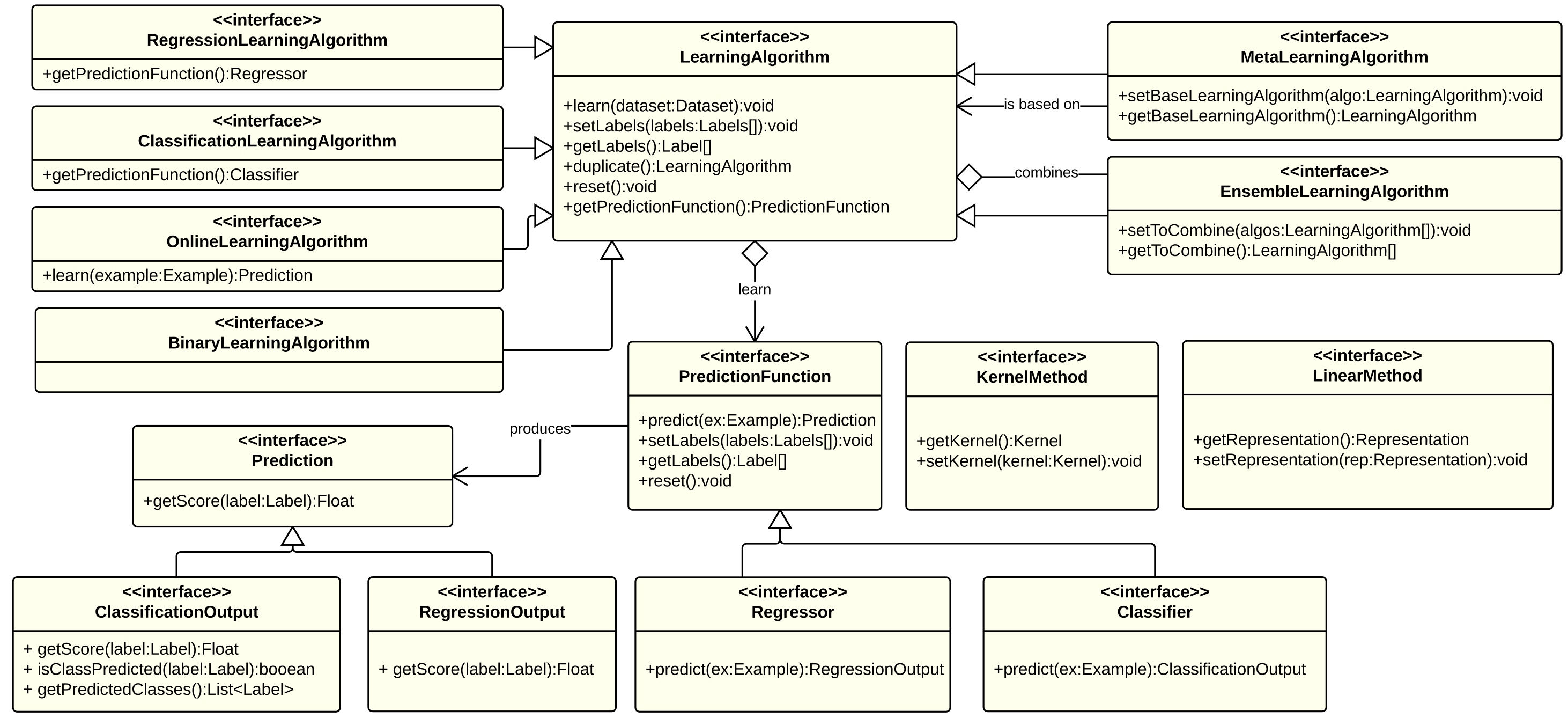February 13, 2017

# Machine Learning Algorithms

In Machine Learning, a wide plethora of learning algorithms have been defined for different purposes, and many variations of the existing ones as well as completely new learning methods are often proposed.
KeLP provides a large number of learning algorithms that can be used to tackle a variety of learning scenarios, including (multi-class, multi-label, sequence) classification, regression and clustering.

Many algorithms are implemented as a linear version to favor efficiency and to allow the exploration of very large datasets; other algorithms have a kernel-based implementation that exploits the expressiveness and efficacy of kernel-methods.
Furthermore KeLP provides both Online Learning (e.g., Perceptron-like learners) and Batch Learning models (e.g., Support Vector Machines). In Batch Learning, the complete training dataset is supposed to be entirely available during the learning phase, and all the examples are exploited at the same time (usually optimizing a global objective function). In Online Learning individual examples are exploited one at a time to incrementally acquire the model.

KeLP proposes a comprehensive set of interfaces to support the implementation of new algorithms. The Figure below illustrates a simplified class diagram of the algorithms in KeLP.Three main classes can be identified:

• LearningAlgorithm: it contains the learning procedure that is applied on training data to generate a PredictionFunction; for instance LibLinearLearningAlgorithm is a binary classification learning algorithm which implements the linear SVM algorithm described in (Fan et al., 2008): given a training dataset it finds the best separating hyperplane between the two classes.
• PredictionFunction: it contains the logic to produce a Prediction on a new data instance. For example, BinaryLinearClassifier classifies a new instance performing a dot product with the classification hyperplane.
• Prediction: it is the output associated to a test data. For instance, BinaryMarginClassifierOutput is the predicted label and score associated to a test data on a binary classification task.

### Existing Learning Algorithms in KeLP

Algorithms are divided w.r.t. the  LearningAlgorithm interfaces they implement, which reflect the learning scenario they solve. Obviously, an algorithm can implement multiple interfaces.

Classification Algorithmsfor algorithms that learn from labeled data how to classify new instances.

• BinaryCSvmClassification
• BinaryNuSvmClassification
• OneClassSvmClassification
• LibLinearLearningAlgorithm
• PegasosLearningAlgorithm
• DCDLearningAlgorithm
• LinearPassiveAggressiveClassification
• KernelizedPassiveAggressiveClassification
• BudgetedPassiveAggressiveClassification
• LinearPerceptron
• KernelizedPerceptron
• SoftConfidenceWeightedClassification

Regression Algorithms: for algorithms that learn from labeled data a regression function.

• EpsilonSvmRegression
• LibLinearRegression
• LinearPassiveAggressiveRegression
• KernelizedPassiveAggressiveRegression

Clustering Algorithms:  for algorithms that are able to organize a dataset into clusters.

• LinearKMeansEngine
• KernelBasedKMeansEngine

Meta-learning Algorithms: for learning approaches that rely on another learning algorithm performing some enrichment, variation, or generalization.

• OneVsAllLearning
• OneVsOneLearning
• MultiLabelClassificationLearning
• MultiEpochLearning
• Stoptron
• RandomizedBudgetPerceptron

Ensemble Algorithms: for algorithms that combine other algorithms in order to provide a more robust solution.

Kernel Methods: for algorithms exploiting kernel functions

• BinaryCSvmClassification (Chang and Lin, 2011)
• BinaryNuSvmClassification (Chang and Lin, 2011)
• OneClassSvmClassification (Chang and Lin, 2011)
• KernelizedPassiveAggressiveRegression
• KernelizedPassiveAggressiveClassification (Crammer et al., 2006)
• BudgetedPassiveAggressiveClassification (Wang and Vucetic, 2010)
• KernelizedPerceptron (Rosenblatt, 1957)
• SoftConfidenceWeightedClassification (Wang et al., 2012)
• KernelBasedKMeansEngine (Kulis et al., 2005)
• Stoptron (Orabona et al., 2008)
• RandomizedBudgetPerceptron (Cesa-Bianchi et al., 2006)

Linear Methods: for algorithms directly operating in an explicit feature space

• LibLinearLearningAlgorithm (Fan et al., 2008)
• LibLinearRegression (Fan et al., 2008)
• PegasosLearningAlgorithm (Shalev-Shwartz et al., 2007)
• DCDLearningAlgorithm (Hsieh et al., 2008)
• LinearPassiveAggressiveClassification (Crammer et al., 2006)
• LinearPassiveAggressiveRegression (Crammer et al., 2006)
• LinearPerceptron (Rosenblatt, 1957)
• SoftConfidenceWeightedClassification (Wang et al., 2012)
• LinearKMeansEngine (Forgy, 1965)

### References

Nicolo’ Cesa-Bianchi and Claudio Gentile. Tracking the best hyperplane with a simple budget perceptron. In Proc. Of The Nineteenth Annual Conference On Computational Learning Theory, pages 483–498. Springer-Verlag, 2006.

Koby Crammer, Ofer Dekel, Joseph Keshet, Shai Shalev-Shwartz, and Yoram Singer. On-line passive-aggressive algorithms. Journal of Machine Learning Research, 7:551–585, December 2006. ISSN 1532-4435.

R.-E. Fan, K.-W. Chang, C.-J. Hsieh, X.-R. Wang, and C.-J. Lin. LIBLINEAR: A library for large linear classification Journal of Machine Learning Research 9(2008), 1871-1874.

E.W. Forgy, Cluster analysis of multivariate data: efficiency versus interpretability of classifications. Biometrics (1965).. 21: 768–769.

Hsieh, C.J., Chang, K.W., Lin, C.J., Keerthi, S. S. and Sundararajan, S. (2008). A Dual Coordinate Descent Method for Largescale Linear SVM. Proceedings of the 25th international conference on Machine learning ICML ’08 (pp. 408415). New York, New York, USA: ACM Press.

Brian Kulis, Sugato Basu, Inderjit Dhillon, and Raymond Mooney. Semi-supervised graph clustering: A kernel approach. In Proceedings of the ICML 2005, pages 457–464, New York, NY, USA, 2005. ACM.

Francesco Orabona, Joseph Keshet and Barbara Caputo. The projectron: a bounded kernelbased perceptron. In Int. Conf. on Machine Learning (2008)

F. Rosenblatt. The Perceptron – a perceiving and recognizing automaton. Report 854601, Cornell Aeronautical Laboratory (1957)

S. Shalev-Shwartz, Y. Singer, and N. Srebro. Pegasos: Primal estimated sub–gradient solver for SVM. In Proceedings of the International Conference on Machine Learning, 2007.

Wang, J., Zhao, P. and Hoi, S.C.. Exact soft confidenceweighted learning. In Proceedings of the ICML 2012. ACM, New York, NY, USA (2012)

Zhuang Wang and Slobodan Vucetic. Online PassiveAggressive Algorithms on a Budget. JMLR W&CP 9:908-915, 2010